Online Education In this page, we are providing Work, Power And Energy Class 9 Extra Questions and Answers Science Chapter 11 pdf download. NCERT Extra Questions for Class 9 Science Chapter 11 Work, Power And Energy with Answers will help to score more marks in your CBSE Board Exams.

## Online Education for Class 9 Science Chapter 11 Extra Questions and Answers Work, Power And Energy

Extra Questions for Class 9 Science Chapter 11 Work, Power And Energy with Answers Solutions

### Work, Power And Energy Class 9 Extra Questions Very Short Answer Type

Work And Energy Class 9 Extra Questions Question 1.
Define the following terms.
(a) Work was done
(b) Energy
(c) Mechanical energy
(d) Kinetic energy
(e) Potential energy
(f) Power
(g) Commercial unit of energy.
(a) Work done: Work done by a force acting on an object is equal to the magnitude of the force multiplied by the distance moved in the direction of the force.
(b) Energy: Energy of a body is defined as the capacity or ability of the body to do work.
(c) Mechanical energy: Mechanical energy includes kinetic energy and potential energy.
(d) Kinetic energy: The energy possessed by a body by virtue of its motion.
(e) Potential energy: The energy possessed by a body due to its position or configuration.
(f) Power: Power is defined as the rate of doing work or the rate of transfer of energy.
(g) Commercial unit of energy: The energy used in households, industries, and commercial establishment are usually expressed in kilowatt-hour.
1 kWh 1 unit = 3.6 x 106J

Work And Energy Class 9 Extra Questions Numericals Question 2.
Write down the type of energy stored in
(a) spring of a watch
(b) flowing water
(c) rolling stone
(d) raised hammer
(e) running athlete
(a) potential energy
(b) kinetic energy
(c) kinetic energy
(d) potential energy
(e) kinetic energy.

Work And Energy Class 9 Important Questions And Answers Question 3.
What will be the kinetic energy of a body when its mass is made four-time and the velocity is doubled?
Initial kinetic energy,
$$E_{K_{i}}=\frac{1}{2} m v^{2}$$
Final kinetic energy,
$$E_{K_{f}}=\frac{1}{2}(4 m) \times(2 v)^{2}$$
= 16 x $$\frac {1}{2}$$mυ2
$$E_{K_{f}}=16 E_{K_{i}}$$

Class 9 Science Chapter 11 Extra Questions Question 4.
If we lift a body of 7 kg vertically upwards to a height of 10 m, calculate the work done in lifting the body.
Given, m = 7 kg
s = 10m
Workdone, W = F x s
E = mg x s
W = 7 x 10 x 10 J
w = 7000 J

Class 9 Work And Energy Extra Questions Question 5.
State the transformation of energy that takes place when

• Green plants prepare their food.
• Head of a nail hammered hard and it becomes hot.

• Solar energy of sun into chemical energy.
• The kinetic energy of the hammer into heat energy.

Extra Questions Of Work And Energy Class 9 Question 6.
How much work is done by a man who tries to push the wall of a house but fails to do so?
W = Fs = 0
As there is no displacement.

Work And Energy Class 9 Extra Questions And Answers Question 7.
Establish a relationship between SI unit and commercial unit of energy.
SI unit of energy is joule and the commercial unit of energy is the joule.
1kWh = 1000 W x 3600 s = 3.6 x 106J

Questions On Work Energy And Power For Class 9 Question 8.
Write down the energy transformation taking place
(a) In electric bulb
(b) In torch
(c) In the thermal power station
(d) In solar cell
(e) Electric heater
(a) Electricity into light energy
(b) The chemical energy of the cell into light and heat energy
(c) The chemical energy of fuel into electricity
(d) Solar energy into electricity
(e) Electricity into heat energy.

Class 9 Science Chapter 11 Extra Questions And Answers Question 9.
A body of mass m is moving in a circular path of radius r. How much work is done on the body?
Zero. This is because the centripetal force acting on the body is perpendicular to the displacement of the body.

Extra Questions On Work And Energy Class 9 Question 10.
A horse of mass 200 kg and a dog of mass 20 kg are running at the same speed. Which of the two possesses more kinetic energy? How?
The kinetic energy of the horse is more as kinetic energy is directly proportional to mass.

Class 9 Science Ch 11 Extra Questions Question 11.
What is the condition for work done to be positive?
For positive work, the angle between force and displacement should be acute.

Class 9 Science Work And Energy Extra Questions Question 12.
Write down the relation between kinetic energy and momentum of a body.
$$E_{K}=\frac{p^{2}}{2 m}$$
Ek = kinetic energy of a body
p = momentum of the body
m = mass of the body.

Work Energy And Power Class 9 Extra Questions Question 13.
A cyclist comes to a skidding stop at 50 m. During this process, the force on the cycle due to the road is 1000 N and is directed opposite to the motion. How much work does the road do on the cycle?
Given,
Displacement, s = 50 m
Force, F = – 1000 N
Workdone, W = F x s
W = -1000 x 50J
W = 50000J

Class 9 Physics Work And Energy Extra Questions Question 14.
A boy pushes a book by applying a force of 40 N. Find the work done by this force as the book is displaced through 25 cm along the path.
Given, Force (F) = 40 N
Displacement (s) = 25 cm = x 10-2 m
Workdone, W = F x s
= 40 x 25 x 10-2
= 10J
∴ W = 10J

### Work, Power And Energy Class 9 Extra Questions Short Answer Type 1

Work And Energy Class 9 Questions Question 1.
State law of conservation of energy and law of conservation of mechanical energy.
Law of conservation of energy: Energy can neither be created nor be destroyed, it can only be transformed from one form to another.
Conservation of mechanical energy: If there is no energy, then the mechanical energy of a system is always constant.

Work And Energy Class 9 Important Questions Question 2.
Define (a) 1 joule (b) 1 watt.
(a) 1 joule is the amount of work done on an object when a force of 1 N displaces it by 1 m along the line of action of the force.
(b) 1 watt is the power of an agent, which does work at the rate of 1 joule per second.

Ch 11 Science Class 9 Extra Questions Question 3.
Write down SI unit of the following quantities.
(a) work
(b) kinetic energy
(c) potential energy
(d) power
(a) joule (J)
(b) joule (J)
(c) joule (J)
(d) watt (W).

Work And Energy Class 9 Extra Numericals Question 4.
What is the sequence of energy change that takes place in the production of electricity from adam?
The potential energy of stored water is converted into the rotational kinetic energy of turbine blades. The rotational kinetic energy of turbine blades is finally converted into electric energy by the generator.

Work Power And Energy Class 9 Extra Questions Question 5.
A light and a heavy object have the same momentum. Find out the ratio of their kinetic energies. Which one has larger kinetic energy?
The relation between kinetic energy and momentum
Given,Question 6.
Why a man does not do work when he moves on a level road while carrying a box on his head?
When a man carries a load on his head, the angle between displacement (s) and force (F) is 900. Therefore work done is zero.

Work, Power And Energy Class 9 Extra Questions Numericals

Question 7.
If an electric iron of 1200 W is used for 30 minutes every day, find electric energy consumed in the month of April.
Given,
Power, P = 1200 W
time, t = 30 minutes
Power, p = $$\frac {W}{t}$$ = $$\frac {E}{t}$$
E = P x t
Energy consumed, E = 1200 x 30 x 60
= 2.16 x 106J = 2.16MJ

Question 8.
What is work done by a force of gravity in the following cases?
(a) Satellite moving around the Earth in a circular orbit of radius 35000 km.
(b) A stone of mass 250 g is thrown up through a height of 2.5 m.
(a) Zero
(b) Given,
mass (m) = 250 g = 0.25 kg
height (h) = 2.5 m
Workdone, W = Fs = mgh
= 0.25 x 10 x 2.5
= 6.25 J
W = 625 J

Question 9.
A car and a truck have kinetic energies of 8 x 10 J and 9 x 10 J respectively. If they are brought to a halt at the same distance, find the ratio of the force applied to both the vehicles.
Given,
Kinetic energy of car, $$\frac {1}{2}$$ = 8 x 10 J
Kinetic energy of truck, $$\frac {1}{2}$$ = 9 x 10 J
W = Fs
Work done,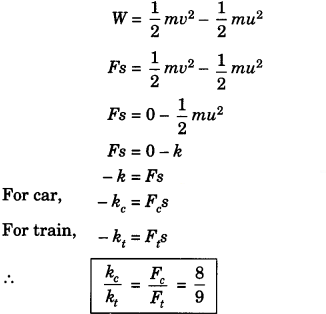Question 10.
A bus of mass 10,000 kg is moving with a velocity 90 km/h. Calculate the work done to stop this bus.
Given,
mass of object, m = 1000 kg
Initial velocity of object, u = 90 km/h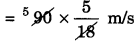= 25 m/s
Work done = $$\frac {1}{2}$$ mυ2 – $$\frac {1}{2}$$ mu2 = 0 – $$\frac {1}{2}$$ x 10000 x (25)2 = 312.5 x 104 J

### Work, Power And Energy Class 9 Extra Questions Short Answer Type 2

Question 1.
The velocity of a body moving in a straight line is inereásed by applying constant force F, for some distance in the direction of motion. Prove that the increase ¡n the kinetic energy of the body is equal to the work done by the force on the body.
Let us consider an object lying on a frictionless surface having mass ‘m’.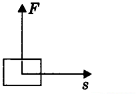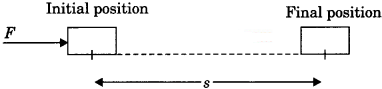A force of constant magnitude ‘F’ is acting on the body. Here initial velocity of the body is u and the final velocity is u. As there is no dissipative forces, work on the body will be stored in the form of a change in kinetic energy. It can be proved as
W = Fs …………(1)
and hence from the equation of motion
υ2 – u2 = 2as
s = $$\frac{v^{2}-u^{2}}{2 a}$$ ………….(2)
Asweknowthat F = ma
Using (1), (2) and (3),
W = ma x $$\frac{v^{2}-u^{2}}{2 a}$$
= $$\frac {1}{2}$$ m (υ2 – u2)
= $$\frac {1}{2}$$mυ2 – $$\frac {1}{2}$$mu2
= change in kinetic energy

Question 2.
Derive an expression for potential energy. Write Its SI unit.
When work is done on the body, the work is stored in the form of energy. Consider an object of mass, m. Let it be raised through a height, h from the ground. A force is required to do this. The minimum force required to raise the object is equal to the weight of the object, mg. The object gains energy equal to the work done on it. Let the work done on the object against gravity h be W.
i.e., W = force x displacement = mgh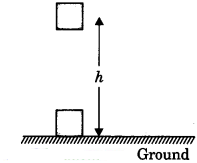Since work done on the object is equal to mgh, an energy equal to mgh units is gained by the object. This is the potential energy (Ep) of the object.
∴ Ep = mgh
SI unit of potential energy is the joule (J).

Work, Power And Energy Class 9 Extra Questions Numericals

Question 3.
A girl having a mass of 35 kg sits on a trolley of mass 5 kg. The trolley is given an initial velocity of 4 ms1 by applying a force. The trolley comes to rest after traversing a distance of 16 m.
(a) How much work is done on the trolley?
(b) How much work is done by the girl?
Given, mass of girl, m = 35 kg
mass of trolley, m = 5 kg.
initial velocity of trolley, u = 4 m/s

(a) using work done = change in kinetic energy
W = $$E_{K_{f}}-E_{K_{i}}$$
= o – $$\frac {1}{2}$$ x 40 x (4)2 = – 320
∴ W = 320 J_J
(b) Work done by the girl = 0.

Question 4.
Express kilowatt in terms of joule per second. A 150 kg car engine develops 500 W for each kg. What forces does it exert in moving the car at a speed of 20 ms-1?
1 kW = iooo w = 103 J/S
Given, mass (m) = 150 kg
power (P) = 500 W
velocity (u) = 20 m/s
Using P = Fυ
or, 500 = F x 20
= F =$$\frac {500}{20}$$ = 25
F= 25 N

Question 5.
How is the power related to the speed at which a body can be lifted? How many kilogrims will a man working at the power of 100 W, be able to lift at constant speed of 1 mr1 vertically? (g = 10 ms-2)
We know that,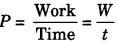or, p = $$\frac {F.s}{t}$$ P = Fυ = mg.υ [F = mg]
where, F = force
s = displacement
υ = velocity
t = time
Given,
power (P) = 100 W
velocity (υ) = 1 m/s
g = 10 m/s2
or, 100 = m x 10 x 1
∴ m = 10 kg

Question 6.
A car of mass 2000 kg is lifted up a distance of 30 m by a crane in 1 minute. A second crane does the same job in 2 minutes. What is the power applied by each crane?
Given, mass of the car to be lifted, m = 2000 kg
height through which the car is to be lifted, h = 30 m
Time taken by first crane, t1 = 1 minute = 60 s
time taken by second crane, t1 = 2 minutes = 120 s
Amount of work done by each crane,
W = mgh = 2000 x 10 x 30 J
W= 6 x 106 J
Power of first crane, ‘
P1 = $$\frac{W_{1}}{t_{1}}=\frac{6 \times 10^{5} \mathrm{J}}{60 \mathrm{s}}$$ = 10kW
Power of second crane
P2 = $$\frac{W_{2}}{t_{2}}=\frac{6 \times 10^{5} \mathrm{J}}{120 \mathrm{s}}$$ = 10kW

Question 7.
Calculate the electricity bill amount for a month of June, if 6 bulbs of 100 W for5 hours, 4 tube lights of 60 W for 5 hours, a TV of 50 W for 6 hours are used per day. The cost per unit is ₹ 5.
Total energy used in a day = (6 x 100 x 5 + 4 x 60 x 5 + 1 x 50 x 6) Wh
= (3000+ 1200 +300)Wh = 4500 Wh
Total energy = 4.5 kWh = 4.5 unit
Total energy used in 30 days = 4.5 x 30 = 135 units
Bill amount = 135 units x ₹ 5 unit = ₹ 675.

### Work, Power And Energy Class 9 Extra Questions Long Answer Type

Question 1.
State the conditions for positive, negative, and zero work. Give at least one example of each.
1. Zero work: If the angle between force and displacement is 90°, then work done is said to be zero work.
Example: When a man carries a load on his head and moves on a level road. Work done by the man on the load is zero.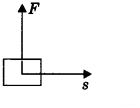2. Positive work: Work done is said to be positive if the force applied on an object and displacement are in the same direction.
W = FsExample: Work done by the force of gravity on a falling body is positive.
3. Negative work: Work done is said to be negative if the applied force on an object and displacement is in opposite direction.
W = -Fs
Her displacement is taken to be negative (- s).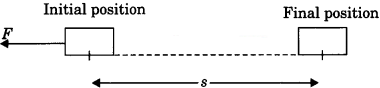Example: Work done by friction force applied is negative on a moving body.

Question 2.
Give a reason for the following:
(a) A bullet is released on firing the pistol.
(b) An arrow moves forward when released from the stretched bow.
(c) Winding the spring of a toy car makes it to run on the ground.
(d) Falling water from a dam generates electricity.
(e) Winding the spring of our watch, the hands of the watch movement.
(a) The chemical energy of gun powder is converted into kinetic energy of the bullet.
(b) The elastic potential energy in a stretched bow is converted into kinetic energy of the arrow.
(c) The potential energy of a spring is converted into kinetic energy of the toy.
(d) The kinetic energy of water is converted into electric energy.
(e) The potential energy of spring due to its windings is converted into mechanical energy of the watch.

Question 3.
State the law of conservation of energy. Show that the energy of a freely falling body is conserved.
Energy can neither be created nor be destroyed, it can only be transformed ‘ m A from one form to another. The total energy before and after the transformation always remains constant.
Let us consider an object of mass ‘m’ dropped from a height h.
Total energy at point A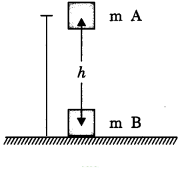$$E_{T_{A}}=E_{K}+E_{P}$$
or, $$E_{T_{A}}=0+m g h$$
∴ $$E_{T_{A}}=m g h$$
Total energy at point B,
$$E_{T_{B}}=E_{T}+E_{p}$$
For finding out velocity at point B
apply υ2 – u2 = 2as
$$v_{\mathrm{B}}^{2}=2 g h$$ = 2gh
Hence, $$E_{T_{B}}=\frac{1}{2} m \mathrm{V}_{\mathrm{B}}^{2}+m g$$
$$E_{T_{B}}=\frac{1}{2} m(2 g h)=m g h$$
Here, $$E_{T_{A}}=E_{T_{B}}$$
Hence if there is no energy loss, total energy is conserved.

### Work, Power And Energy Class 9 Extra Questions HOTS

Question 1.
A running man has half the kinetic energy that a body of half of his mass has. The man speeds up by 1 m/s and then has the same kinetic energy as the boy. What are the original speeds of the man and the boy?
Let us take
mass of boy = m
mass of man = M
velocity of boy = u
velocity of man = υ
Here, m = $$\frac {M}{2}$$
Initially
EKof man = $$\frac {1}{2}$$ EK of boy
$$\frac {1}{2}$$ Mυ2 = $$\frac {1}{2}$$ mu2 x $$\frac {1}{2}$$
and $$\frac {1}{2}$$ Mυ2 = $$\frac {1}{2}$$ $$\frac{1}{2}\left(\frac{M}{2}\right) u^{2} \times \frac{1}{2}$$
∴ υ2 = $$\frac{u^{2}}{4}$$ ………….(1)

Finally
EK of man = EK of boy
$$\frac {1}{2}$$M(υ +1)2 = $$\frac {1}{2}$$ mu2
$$\frac {1}{2}$$M(υ +1)2 = $$\frac{1}{2}\left(\frac{M}{2}\right) u^{2}$$
(υ +1)2 = $$\frac{u^{2}}{2}$$
∴ υ +1 = $$\frac{u}{\sqrt{2}}$$ …………(2)
υ = $${\sqrt{2}}$$ + 1 = 2.41 m/s
and u = 4.82 m/s

Question 2.
Avinash can run with a speed of 8 m/s against the frictional force of 10 N, and Kapil can move with a speed of 3 m/s against the frictional force of 25 N. Who is more powerful and why?
Power can be expressed as, P = Fu
Power of Avinash, P = Fu = 10 x 8 = 80 W
Power of Kapil, P = Fu = 25 x 3 = 75 W
Avinash is more powerful than Kapil.

Question 3.
The weight of a person on a planet A is about half that on the earth. He can jump up to 0.4 m height on the surface of the earth. How high can he can jump on planet A?
For the case, of jump, the energy imparted by the person is converted into potential energy,
Hence,
(mAgA)hA = (mege)he ………(1)
Given, mAgA = $$\frac{m_{e} g_{e}}{2}$$ ………….(2)
Using (1) and (2),
$$\frac{h_{\mathrm{A}}}{2}=h_{e}$$
∴ hA = 2he = 2 x 0.4 = 0.8m

Question 4.
A ball is dropped from a height of 10 m. If the energy of the bal] is reduced by 40% after striking the ground, how much high can the ball bounce back? (g 10 m/s2)
Given,
height, h = 10
If the energy of the ball is reduced by 40%, the remaining energy of the ball is 60% of initial.
Hence ball will rebound to 60% of the initial height
h = $$\frac {60}{100}$$ x 10 m
∴ h = 6m

Question 5.
Four men lift a 250 kg box to a height of 1 m and hold it. Without raising or lowering it
(a) How much work is done by men in lifting the box?
(b) How much work they do in just holding it?
Given, mass of block, m = 250 kg
height, h = 1 m
(a) work done in lifting,
W = Fs = mgh = 250 x 10 x 1
W = 2500 J
(b) work done in holding, W = 0

Question 6.
What is power? How do you differentiate kilowatt from kilowatt-hour? The Jog falls in Karnataka state are nearly 20 m high. 2000 tonnes of water falls from it in a minute. Calculate the equivalent power if all this energy can be utilized. (g = 10 ms-2)
1. Power is rate of doing work.
2. Kilowatt is the unit of power and kilowatt-hour is unit of energy.
3. Given, height, h = 20 m
Mass per unit time,
m/t = 2000 tonnes per minutes = $$\frac{2000 \times 10^{3}}{60}$$ kg/s
Power P = $$\frac{W}{t}=\frac{m g h}{t}=\frac{2000 \times 10^{3}}{60}$$ = 10 x 20
∴ P = 6.67 x 106 W = 6.67 MW

Question 7.
What happens to the kinetic energy when:
1.  the mass of the body is doubled at constant velocity?
2. the velocity of the body is doubled at constant mass?
3. the mass of the body is doubled but velocity is reduced to one fourth?
1. The kinetic energy of body is given by,
Ek = $$\frac {1}{2}$$ mυ6, Ek ∝ m. If the mass of the body is doubled its kinetic energy is also doubled.

2. Kinetic energy, Ek ∝ u2
If velocity of the body is doubled, its kinetic energy becomes four times.

3. Initial kinetic energy,
$$E_{K_{i}}$$= $$\frac {1}{2}$$ mu2
Final kinetic energy,
$$E_{K_{f}}$$ = $$\frac {1}{2}$$(2m) $$\left(\frac{v}{4}\right)^{2}=\frac{m v^{2}}{16}$$
Kinetic Energy becomes one eighth.

Question 8.
Why is the water at the bottom of a waterfall warmer than the water on the top?
When waterfalls, its potential energy is converted into kinetic energy of molecules, and the kinetic energy of molecules is converted into heat energy.

### Work, Power And Energy Class 9 Extra Questions Value Based (VBQs)

Question 1.
Aman is a student of class IX. He saw an old man trying to keep his box on the roof of a bus but was unable to do so. Aman picked up his box and placed the box on the roof of the bus.
The old man thanked Aman.
Answer the following questions based on the given paragraph:

• Is the work done by Aman while placing the box on the roof of the bus positive or negative?
• Is the work done by gravity on the box positive or negative?
• What values are shown by Aman?

• Positive
• Negative
• Aman is a kind and helpful person.

Question 2.
In the winter season, John gifted an electric heater to his grandfather. The electric heater uses electricity to increase room temperature.
Answer the following questions based on the above paragraph:

• Write down the energy transformation in the electric heater.
• What values are shown by John?